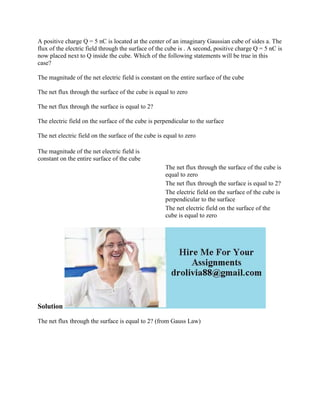Successfully reported this slideshow.

# A positive charge Q - 5 nC is located at the center of an imaginary Ga.docx×

# A positive charge Q - 5 nC is located at the center of an imaginary Ga.docx

A positive charge Q = 5 nC is located at the center of an imaginary Gaussian cube of sides a. The flux of the electric field through the surface of the cube is . A second, positive charge Q = 5 nC is now placed next to Q inside the cube. Which of the following statements will be true in this case?
The magnitude of the net electric field is constant on the entire surface of the cube
The net flux through the surface of the cube is equal to zero
The net flux through the surface is equal to 2?
The electric field on the surface of the cube is perpendicular to the surface
The net electric field on the surface of the cube is equal to zero
Solution
The net flux through the surface is equal to 2? (from Gauss Law)
.

A positive charge Q = 5 nC is located at the center of an imaginary Gaussian cube of sides a. The flux of the electric field through the surface of the cube is . A second, positive charge Q = 5 nC is now placed next to Q inside the cube. Which of the following statements will be true in this case?
The magnitude of the net electric field is constant on the entire surface of the cube
The net flux through the surface of the cube is equal to zero
The net flux through the surface is equal to 2?
The electric field on the surface of the cube is perpendicular to the surface
The net electric field on the surface of the cube is equal to zero
Solution
The net flux through the surface is equal to 2? (from Gauss Law)
.